Please wait a minute...
 Chin. Phys. B, 2020, Vol. 29(11): 116701    DOI: 10.1088/1674-1056/abbbe8
 CONDENSED MATTER: STRUCTURAL, MECHANICAL, AND THERMAL PROPERTIES Prev   Next

# Ground-state phases and spin textures of spin–orbit-coupled dipolar Bose–Einstein condensates in a rotating toroidal trap

Qing-Bo Wang(王庆波)1,2, Hui Yang(杨慧)1,3, Ning Su(苏宁)1, and Ling-Hua Wen(文灵华)1, †
1 Key Laboratory for Microstructural Material Physics of Hebei Province, School of Science, Yanshan University, Qinhuangdao 066004, China
2 School of Physics and Technology, Tangshan Normal University, Tangshan 063000, China
3 Department of Physics, Xinzhou Teachers University, Xinzhou 034000, China
 Abstract  We investigate the ground-state phases and spin textures of spin–orbit-coupled dipolar pseudo-spin-1/2 Bose–Einstein condensates in a rotating two-dimensional toroidal potential. The combined effects of dipole–dipole interaction (DDI), spin–orbit coupling (SOC), rotation, and interatomic interactions on the ground-state structures and topological defects of the system are analyzed systematically. For fixed SOC strength and rotation frequency, we provide a set of phase diagrams as a function of the DDI strength and the ratio between inter- and intra-species interactions. The system can show rich quantum phases including a half-quantum vortex, symmetrical (asymmetrical) phase with quantum droplets (QDs), asymmetrical segregated phase with hidden vortices (ASH phase), annular condensates with giant vortices, triangular (square) vortex lattice with QDs, and criss-cross vortex string lattice, depending on the competition between DDI and contact interaction. For given DDI strength and rotation frequency, the increase of the SOC strength leads to a structural phase transition from an ASH phase to a tetragonal vortex lattice then to a pentagonal vortex lattice and finally to a vortex necklace, which is also demonstrated by the momentum distributions. Without rotation, the interplay of DDI and SOC may result in the formation of a unique trumpet-shaped Bloch domain wall. In addition, the rotation effect is discussed. Furthermore, the system supports exotic topological excitations, such as a half-skyrmion (meron) string, triangular skyrmion lattice, skyrmion–half-skyrmion lattice, skyrmion–meron cluster, skyrmion–meron layered necklace, skyrmion–giant-skyrmion necklace lattice, and half-skyrmion–half-antiskyrmion necklace. Keywords:  Bose-Einstein condensate      dipole-dipole interaction      spin-orbit coupling      topological defects Received:  29 June 2020      Revised:  17 August 2020      Accepted manuscript online:  28 September 2020 Fund: the National Natural Science Foundation of China (Grant Nos. 11475144 and 11047033), the Natural Science Foundation of Hebei Province, China (Grant Nos. A2019203049 and A2015203037), the University Science and Technology Foundation of Hebei Provincial Department of Education, China (Grant No. Z2017056), Science and Technology Plan Projects of Tangshan City, China (Grant No. 19130220g), and Research Foundation of Yanshan University, China (Grant No. B846). Corresponding Authors:  †Corresponding author. E-mail: linghuawen@ysu.edu.cn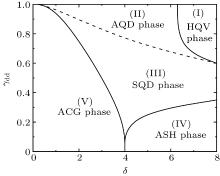Fig. 1.  The γdd − δ phase diagram with γdd > 0, Ω = 0.4, and λ = 1.5. There are five different ground-state phases marked by (I)–(V). (I) half-quantum vortex phase (HQV phase), (II) asymmetrical phase with quantum droplets (AQD phase), (III) symmetrical phase with quantum droplets (SQD phase), (IV) asymmetrical segregated phase with hidden vortices (ASH phase), and (V) annular condensates with giant vortices (ACG phase). The unit length is a0.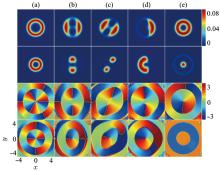Fig. 2.  Density distributions and phase distributions for different repulsive γdd with Ω = 0.4 and λ = 1.5: (a) γdd = 0.5, δ = 1.7, (b) γdd = 0.5, δ = 4, (c) γdd = 0.85, δ = 6, (d) γdd = 0.15, δ = 7.5, and (e) γdd = 0.65, δ = 7.5. The rows from top to bottom represent |ψ1|2, | ψ2|2, argψ1, and argψ2, respectively. The unit length is a0.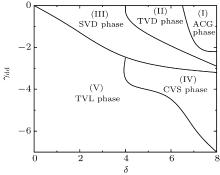Fig. 3.  The γdd−δ phase diagram with γdd < 0, Ω = 0.4, and λ = 1.5. There are five different ground-state phases marked by (I)–(V). (I) annular condensates with giant vortices (ACG phase), (II) triangular vortex lattice with quantum droplets (TVD phase), (III) square vortex lattice with quantum droplets (SVD phase), (IV) criss-cross vortex string lattice (CVS phase), and (V) triangular vortex lattice (TVL phase). The unit length is a0.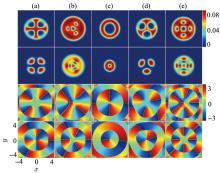Fig. 4.  Density distributions and phase distributions for different attractive γdd with Ω = 0.4 and λ = 1.5: (a) γdd = −3, δ = 7.5, (b) γdd = −6, δ = 4, (c) γdd = −2, δ = 7.5, (d) γdd = −2.5, δ = 7.5, and (e) γdd = −3, δ = 4. The rows from top to bottom represent |ψ1|2, |ψ2|2, arg ψ1, and arg ψ2, respectively. The unit length is a0.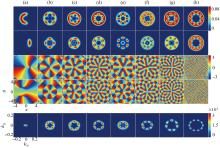Fig. 5.  Density distributions and phase distributions for different isotropic SOC strength λ with Ω = 0.8 and γdd = 0.5: (a) λ = 0, δ = 4, (b) λ = 1.5, δ = 4, (c) λ = 2, δ = 4, (d) λ = 3, δ = 4, (e) λ = 2, δ = 7.5, (f) λ = 3, δ = 7.5, (g) λ = 4, δ = 7.5 and (h) λ = 10, δ = 7.5. The rows from top to bottom represent |ψ1|2, |ψ2|2, argψ1, argψ2, and momentum distribution of component 2, respectively.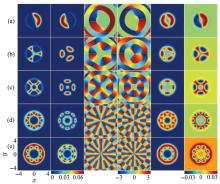Fig. 6.  Ground states of rotating spin-1/2 BECs with SOC and DDI in a toroidal trap, where γdd = 0.5, λ = 2, and δ = 7.5. (a) Ω = 0, (b) Ω = 0.3, (c) Ω = 0.5, (d) Ω = 1, and (e) Ω = 1.4. The rows from left to right represent |ψ1|2, |ψ2|2, arg ψ1, arg ψ2, |ψ1|2 + |ψ2|2, and |ψ1|2 − |ψ2|2, respectively. The unit length is a0.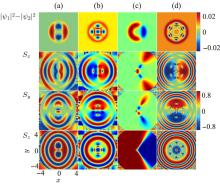Fig. 7.  Density differences and spin densities of rotating spin-1/2 BECs with SOC and DDI in a toroidal trap. The rows from top to bottom denote |ψ1|2−|ψ2|2, Sx, Sy, and Sz, respectively. The corresponding ground states in (a)-(d) are shown in Figs. 2(b), 4(e), 5(a), and 5(d), respectively. The unit length is a0.Table 1.  Typical ground-state phases of two-component BECs.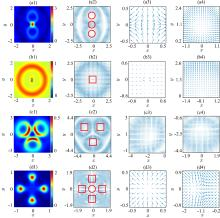Fig. 8.  Topological charge densities and spin textures of spin–orbit-coupled dipolar BECs in a rotating toroidal trap, where the corresponding ground states for (a)–(d) are given in Figs. 2(b), 4(c), 4(d), and 4(e), respectively. The first column (from left to right) represents topological charge density, the second column is spin texture, and the right two columns denote the local amplifications of the spin texture. The red solid square and the red solid circle denote a skyrmion and a half-skyrmion, respectively. The unit length is a0.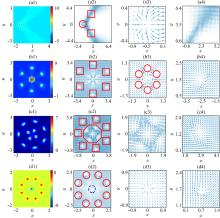Fig. 9.  Topological charge densities and spin textures of spin–orbit-coupled dipolar BECs in a rotating toroidal trap, where the ground states for (a)–(d) are shown in Figs. 5(a), 5(d), 5(f), and 6(e), respectively. The first column (from left to right) denotes topological charge density, the second column is spin texture, and the right two columns represent the local amplifications of the spin texture. The red solid square, red solid circle, red solid octagon, and blue dotted circle represent a skyrmion, a half-skyrmion, a giant skyrmion, and a half-antiskyrmion, respectively. The unit length is a0.